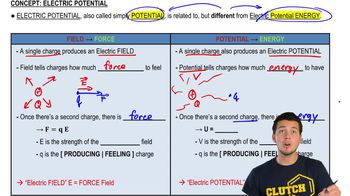Start typing, then use the up and down arrows to select an option from the list.
7:50 minutes
Problem 23f
Textbook Question

# A small particle has charge -5.00 μC and mass 2.00x10^-4 kg. It moves from point A, where the electric po-tential is V_A = +200 V, to point B, where the electric potential is V_B = +800 V. The electric force is the only force acting on the particle. The particle has speed 5.00 m/s at point A. What is its speed at point B? Is it moving faster or slower at B than at A? Explain.Verified Solution
This video solution was recommended by our tutors as helpful for the problem above.
38views